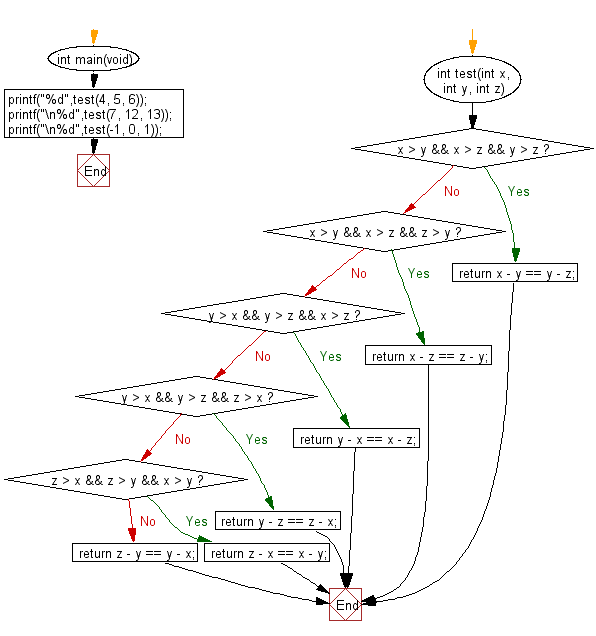﻿ C : Check the difference between small, medium and large# C Exercises: Check three given integers and return true if the difference between small and medium and the difference between medium and large is same

## C-programming basic algorithm: Exercise-33 with Solution

Write a C program to check three given integers (small, medium and large) and return true if the difference between small and medium and the difference between medium and large is same.

C Code:

``````#include <stdio.h>
#include <stdlib.h>
int main(void){
printf("%d",test(4, 5, 6));
printf("\n%d",test(7, 12, 13));
printf("\n%d",test(-1, 0, 1));
}
int test(int x, int y, int z)
{
if (x > y && x > z && y > z) return x - y == y - z;
if (x > y && x > z && z > y) return x - z == z - y;
if (y > x && y > z && x > z) return y - x == x - z;
if (y > x && y > z && z > x) return y - z == z - x;
if (z > x && z > y && x > y) return z - x == x - y;
return z - y == y - x;
}
``````

Sample Output:

```1
0
1
```

Pictorial Presentation:Flowchart:C Programming Code Editor:

What is the difficulty level of this exercise?

Test your Programming skills with w3resource's quiz.

﻿

## C Programming: Tips of the Day

Why doesn't a+++++b work?

printf("%d",a+++++b); is interpreted as (a++)++ + b according to the Maximal Munch Rule!.

++ (postfix) doesn't evaluate to an lvalue but it requires its operand to be an lvalue.

! 6.4/4 says the next preprocessing token is the longest sequence of characters that could constitute a preprocessing token"

Ref : https://bit.ly/3fdldUT